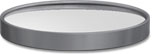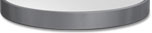Reflection of LightNormal to
Surface
Incident Angle
Reflected Angle
(n = refractive index, Θ = angle)
(Because n = n in a reflection...)
(The n's cancel...)
(Leaving Θ(i) = Θ(r))